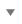# Extend Curve

Extend a curve and edit the extension.

1. On the Geometry ribbon, select the Extend Curve tool.Note: The tool may be hidden in a dropdown menu. To access the dropdown menu, you can do one of the following:
• Click and hold the currently displayed tool (NURBS).
• Selectat the lower right corner of the currently displayed tool (NURBS).
The guide panel appears.
2. Extend a curve:
• Click a curve near the end point. By default, the curve is extended at the end point at a length of 1.0.
• In the guide bar, select one of the following options:
• Start: Extend the curve at the start point.
• End: Extend the curve at the end point.
• Both: Extend the curve at both the start point and end point.
Tip: To display information about the extension, click the end point of the extension.
3. Edit the extension.
To Do this
Change the location of the extension In the guide bar, select one of the following options:
• Start: Extend the curve at the start point.
• End: Extend the curve at the end point.
• Both: Extend the curve at both the start point and end point.
Change the shape In the guide bar, select one of the following options:
• Linear Shape (default): The extension is a segment.
• Soft Shape: The extension has continuous curvature.
• Reflective Shape: The extension is the mirror image of the curve.
• Natural Shape: The original curve is extended.
• Arc Shape: The extension is shaped like an arc.
Change the length of the extension
• Drag the end point of the extension.
• In the guide bar, select Extension Distance, and then enter a Length.
• Click the end point. In the microdialog, select Extension Distance, and then enter a Length.
Change the increment factor The increment factor increases the length by a percentage. For example, if you enter a value of 0.1, the length increases by 10%.
• In the guide bar, select Increment Factor, and then enter a Parameter.
• Click the end point. In the microdialog, select Increment Factor, and then enter a Parameter.
Change the total length of the curve
• In the guide bar, select Total Length, and then enter a Length.
• Click the end point. In the microdialog, select Total Length, and then enter a Length.
Change the parameter distance
• In the guide bar, select Up to Parameter, and then enter a Parameter distance.
• Click the end point. In the microdialog, select Up to Parameter, and then enter a Parameter distance.
Change the reference curve You can extend the curve to another curve.
• In the guide bar, select Reference curve/surface, and then click a curve.
• Click the end point. In the microdialog, select Reference curve/surface, and then click a curve.

This option is indicated by green handles at the end point of the extension.

4. Right-click and mouse through the check mark to exit, or double-right-click.